# 例子

• 下图是二维的欧式密铺 omnitruncated (4, 2, 4)：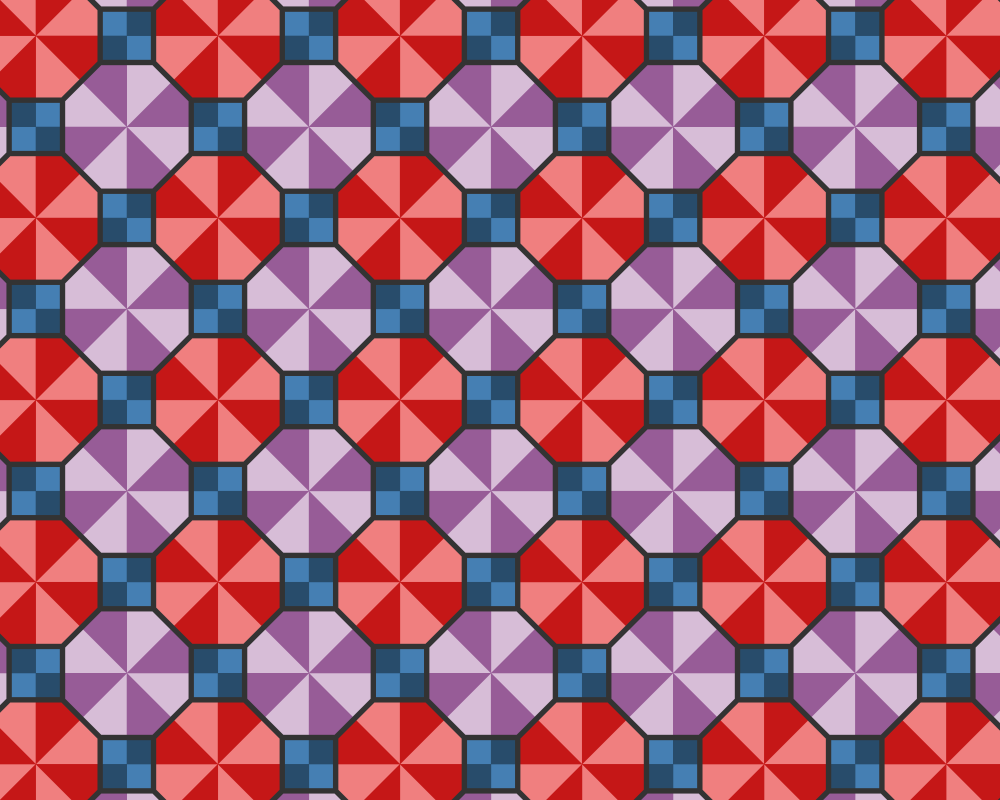• 下图是二维的 Poincaré 圆盘上的正双曲密铺 (2, 3, 13)，绘制了 3447 个顶点，6971 条边，3549 个多边形：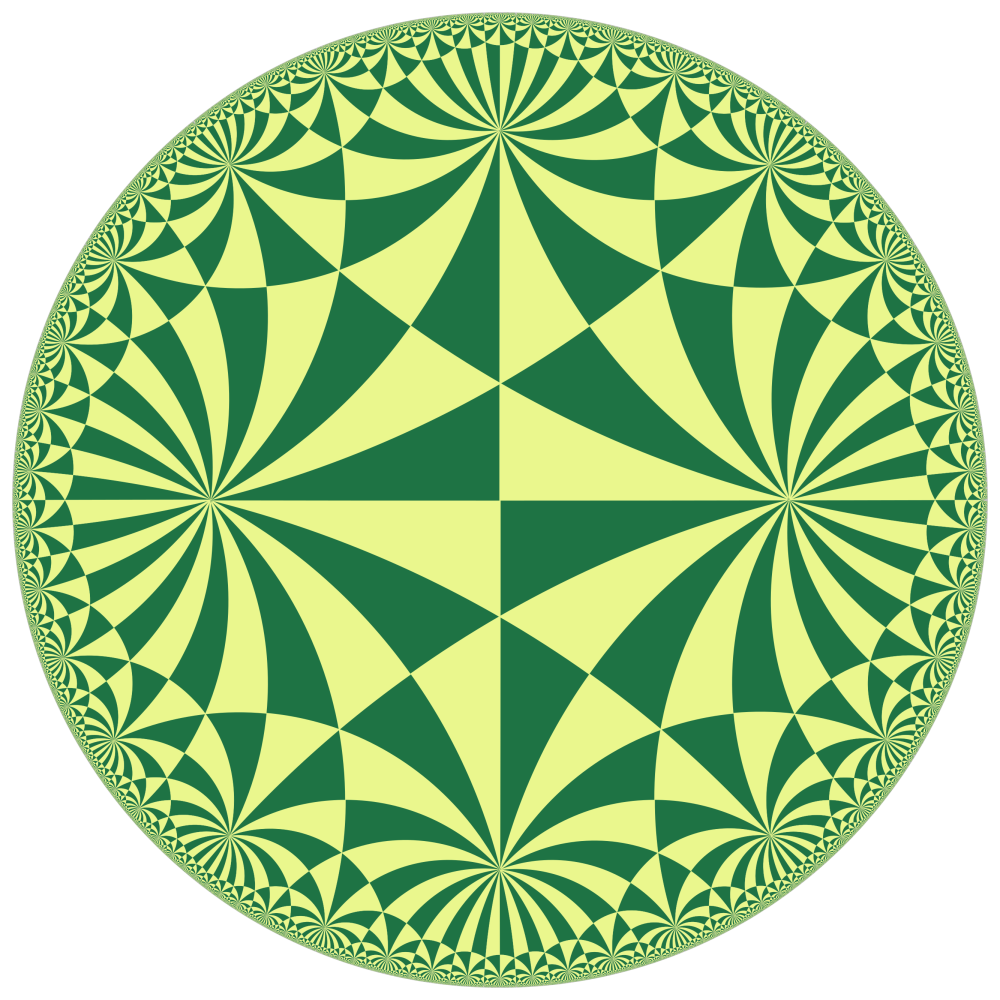• 下图是二维的上半平面模型中的双曲密铺 omnitruncated (4, 3, 3):• 下图是三维的 Poincaré 单位球中的正双曲密铺 (3, 5, 3)：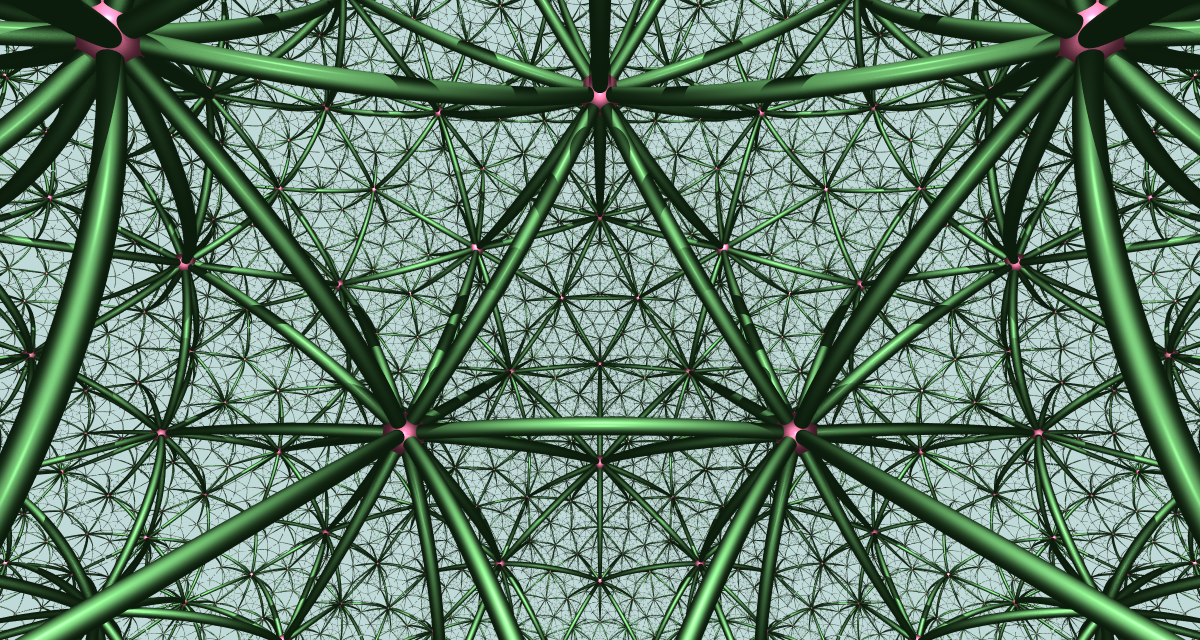• 下图是三维的 Poincaré 单位球中的正双曲密铺 (5, 3, 5)：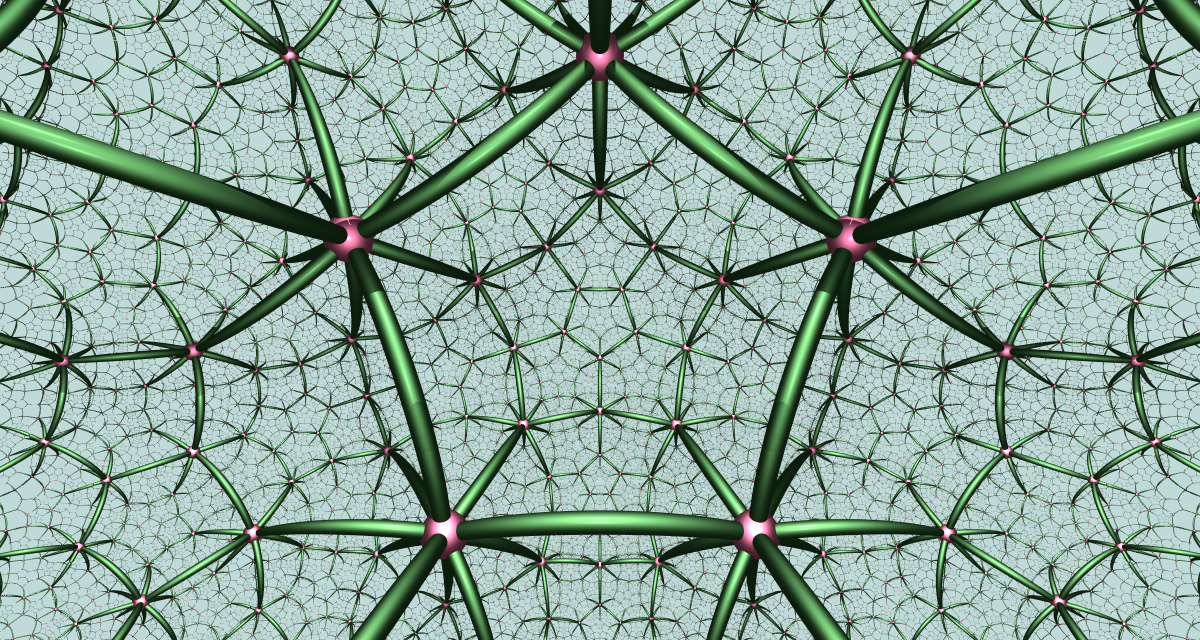• 下图是三维的 Poincaré 单位球中的正双曲密铺 (5, 3, 4)：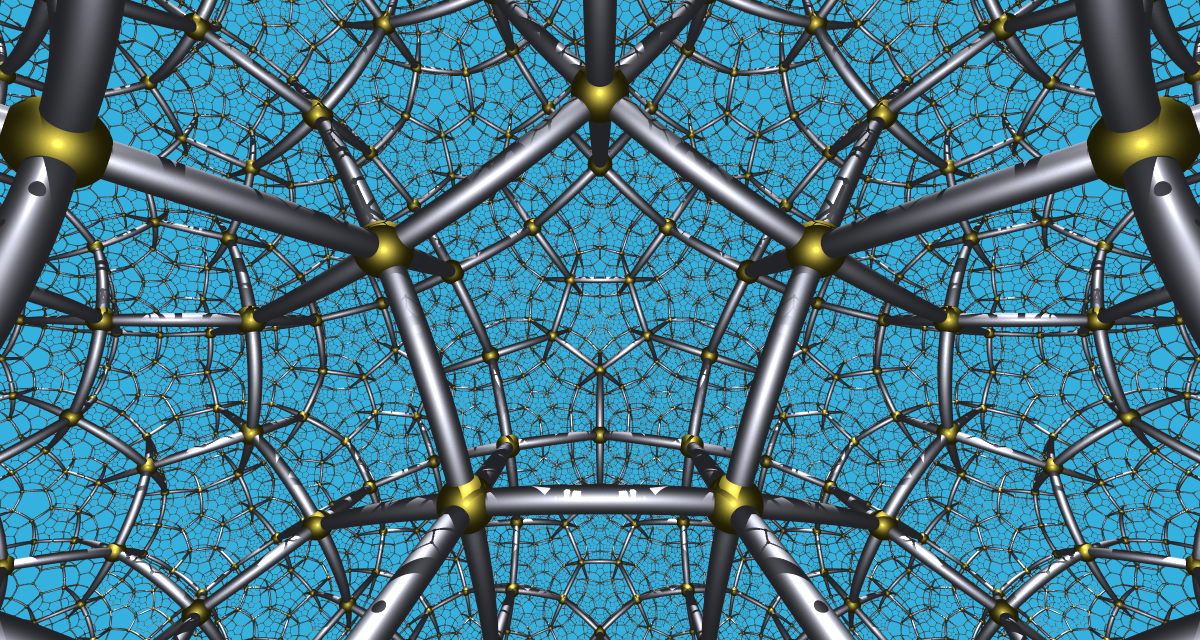• 下图是三维的 Poincaré 单位球中的正双曲密铺 (4, 3, 5)：• 以上四个密铺是三维双曲空间中仅有的正且每个胞腔为紧集的双曲密铺。如果去掉每个胞腔为紧集的限制的话，但是要求每个胞腔的体积有限的话，则还有 10 个正双曲密铺，如下图的 (6, 3, 3)：你可以看到每个胞腔都有一个理想顶点，它位于空间的无穷远处 (即单位球面上)。这 10 个密铺也叫做仿紧的 (paracompact)，因为每个胞腔的体积仍然是有限的。如果再去掉每个胞腔的体积有限的条件的话，得到的密铺叫做非紧的，这样的正密铺有无限多个。

• 如果去掉正密铺这个限制，只考虑均匀密铺的话，那例子就非常多了。比如我们可以从正密铺出发通过截断操作得到许多均匀密铺，如 rectified (3, 5, 3) 和 rectified (5, 3, 4)：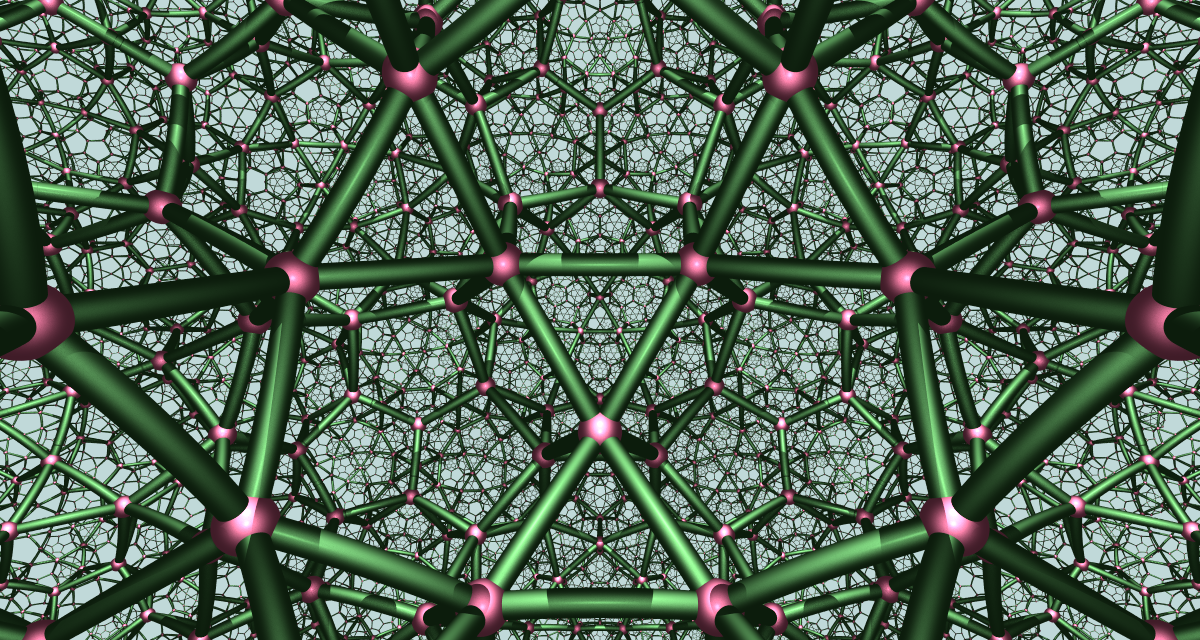还有来自 [5, 31,1] 的密铺 cantic order-5 cubic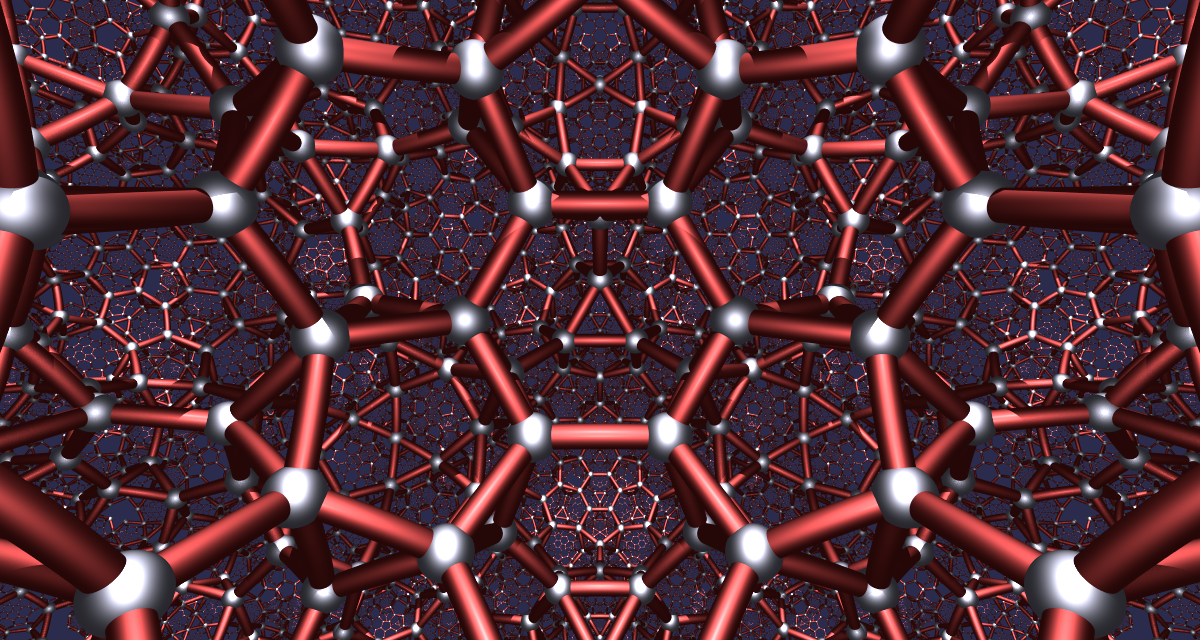• 下图是一个二维的球面密铺 omnitruncated (5, 2, 3)，绘制在了三维的球面上：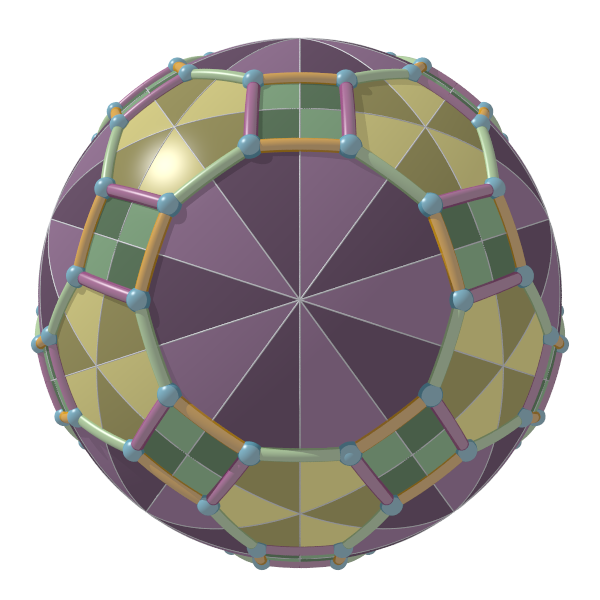• 最后是一个 shader 程序，仍然来自 Matt Zucker 的 shadertoy 项目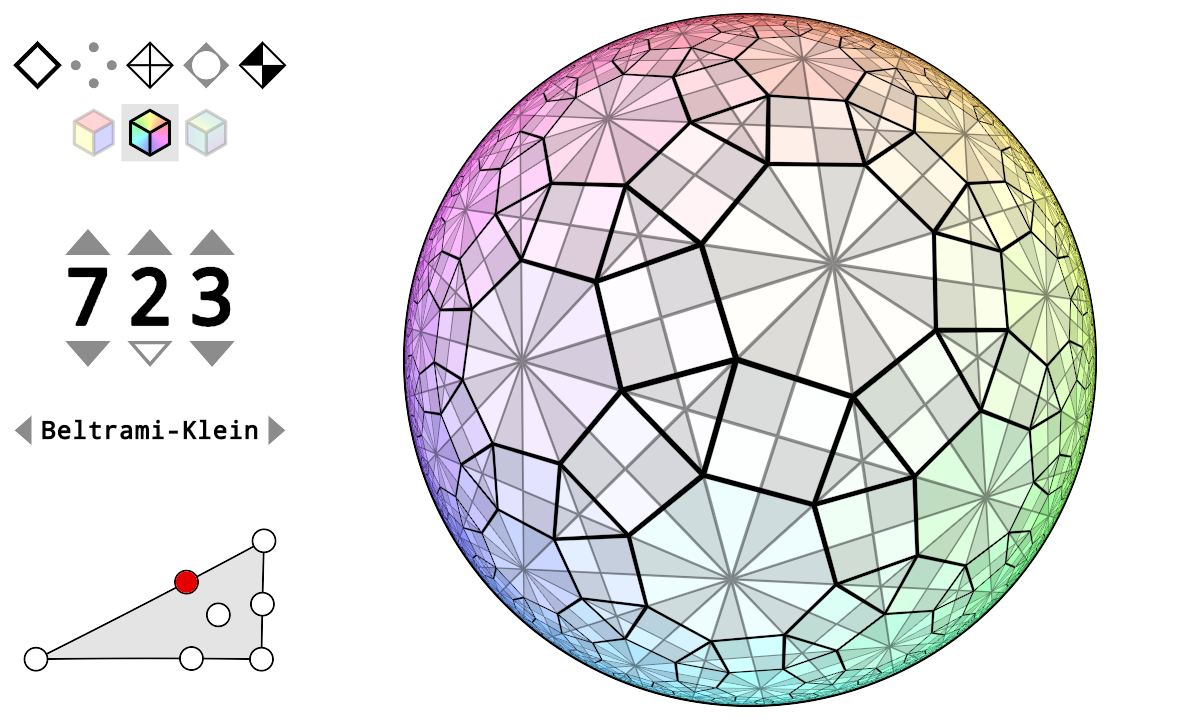# 图像的绘制原理

Wythoff 构造法中最关键的部分在于如何快速地、不重复地计算所有顶点坐标，以及它们之间的边、面、胞腔的关系。这正是这个程序的优雅之处，它采用的是群论的方法，首先在密铺的对称群中进行符号计算，得出每个顶点在最短字典序下对应的单词表示，边的下标，面的下标等信息，然后将每个顶点对应的单词作用在初始顶点上得到该顶点的坐标。换句话说，在计算每个顶点的最终坐标之前，它已经提前计算好了有多少个顶点，每个顶点是由初始顶点经过哪些反射得到的，哪些顶点构成边，哪些顶点构成面，哪些顶点构成胞腔，等等。这些计算仅涉及整数运算，完全避免了浮点数精度损失的问题。是不是很神奇呢？

# 例子：omnitruncated (7, 2, 3) 密铺

Omnitruncated (7, 2, 3) 密铺对应的 Coxeter-Dynkin 图如下：$M=\begin{pmatrix} 1 & 7 & 2 \\ 7 &1 &3\\ 2 & 3 &1\end{pmatrix}$

$W=\langle s_0,s_1, s_2\ |\ s_0^2=s_1^2=s_2^2=(s_0s_1)^7=(s_1s_2)^3=(s_0s_2)^2=1\rangle.$

$W$ 中每个元素 $g$ 都可以表示为生成元 $s_0,s_1,s_2$ 的乘积，我们称任何这样的表示方式为 $g$ 的一个单词表示。$g$ 的单词表示不是唯一的，比如从定义 $W$ 的生成关系中可以看出 $s_0s_2=s_2s_0$, $s_1s_2s_1=s_2s_1s_2$ 等等。但是在 $g$ 的所有单词表示中，我们总是可以选择“最小的”那个来作为 $g$规范表示，这个排序的依据就是最短字典序 (shortlex order)：首先规定生成元 $s_0,s_1,s_2$ 之间的字母顺序关系为 $s_0<s_1<s_2$，然后将这个顺序扩展到任何两个单词 $w_1$$w_2$ 上去：

1. 先比较长度，长度长的为较大者，即若 $n<m$$w_1<w_2$$n>m$$w_1>w_2$
2. 若长度相等则按字母顺序从左到右逐项比较，设 $k$ 使得对任何 $l<k$$s_{i_l}=s_{j_l}$$s_{i_k}\ne s_{j_k}$，则规定 $w_1,w_2$ 之间的大小关系与 $s_{i_k},s_{j_k}$ 之间的大小关系相同。

$\begin{array}{lllll}e&s_{0}&s_{1}&s_{2}&s_{0}s_{1}\\s_{0}s_{2}&s_{1}s_{0}&s_{1}s_{2}&s_{2}s_{1}&s_{0}s_{1}s_{0}\\s_{0}s_{1}s_{2}&s_{0}s_{2}s_{1}&s_{1}s_{0}s_{1}&s_{1}s_{0}s_{2}&s_{1}s_{2}s_{1}\\s_{2}s_{1}s_{0}&s_{0}s_{1}s_{0}s_{1}&s_{0}s_{1}s_{0}s_{2}&s_{0}s_{1}s_{2}s_{1}&s_{0}s_{2}s_{1}s_{0}\\s_{1}s_{0}s_{1}s_{0}&s_{1}s_{0}s_{1}s_{2}&s_{1}s_{0}s_{2}s_{1}&s_{1}s_{2}s_{1}s_{0}&s_{2}s_{1}s_{0}s_{1}\\s_{0}s_{1}s_{0}s_{1}s_{0}&s_{0}s_{1}s_{0}s_{1}s_{2}&s_{0}s_{1}s_{0}s_{2}s_{1}&s_{0}s_{1}s_{2}s_{1}s_{0}&s_{0}s_{2}s_{1}s_{0}s_{1}\\s_{1}s_{0}s_{1}s_{0}s_{1}&s_{1}s_{0}s_{1}s_{0}s_{2}&s_{1}s_{0}s_{1}s_{2}s_{1}&s_{1}s_{0}s_{2}s_{1}s_{0}&s_{1}s_{2}s_{1}s_{0}s_{1}\\s_{2}s_{1}s_{0}s_{1}s_{0}&s_{2}s_{1}s_{0}s_{1}s_{2}&\end{array}$1. 长度为 0 的路径对应的是 $W$ 的单位元。
2. 长度为 1 的三条路径 $0\xrightarrow{\ s_0\ }1$, $0\xrightarrow{\ s_1\ }2$, $0\xrightarrow{\ s_2\ }8$ 对应的是 $W$ 的三个长度为 1 的元素 $s_0,s_1,s_2$
3. 长度为 2 的 5 条路径 $0\xrightarrow{\ s_0\ }1\xrightarrow{\ s_1\ }2$, $0\xrightarrow{\ s_0\ }1\xrightarrow{\ s_2\ }8$, $0\xrightarrow{\ s_1\ }2\xrightarrow{\ s_0\ }3$, $0\xrightarrow{\ s_1\ }2\xrightarrow{\ s_2\ }8$, $0\xrightarrow{\ s_2\ }8\xrightarrow{\ s_1\ }9$ 对应的是 $W$ 的 5 个长度为 2 的元素 $s_0s_1,s_0s_2,s_1s_0,s_1s_2,s_2s_1$$\begin{array}{llll}e&s_{0}&s_{1}&s_{2}\\s_{0}s_{1}&s_{0}s_{2}&s_{1}s_{0}&s_{1}s_{2}\\s_{2}s_{1}&s_{0}s_{1}s_{0}&s_{0}s_{1}s_{2}&s_{0}s_{2}s_{1}\\s_{1}s_{0}s_{2}&s_{1}s_{2}s_{1}&s_{2}s_{1}s_{0}&s_{0}s_{1}s_{0}s_{2}\\s_{0}s_{1}s_{2}s_{1}&s_{0}s_{2}s_{1}s_{0}&s_{1}s_{0}s_{2}s_{1}&s_{1}s_{2}s_{1}s_{0}\\s_{0}s_{1}s_{0}s_{2}s_{1}&s_{0}s_{1}s_{2}s_{1}s_{0}&s_{1}s_{0}s_{2}s_{1}s_{0}&s_{0}s_{1}s_{0}s_{2}s_{1}s_{0}\end{array}$

$w=s_{i_0}s_{i_1}\cdots s_{i_n}$，初始顶点为 $v_0$，则 $w$$v_0$ 上的作用为 $w\cdot v_0 = s_{i_0}(s_{i_1}(\cdots s_{i_n}(v_0)).$ 即从右到左依次计算每个生成元作用的结果。当然由于 $W$ 是无限群，我们只能计算那些长度不超过一定范围的群元素对应的顶点。假设我们已经有了前面这 37 个顶点，把它们按照最短字典序排序存储在一个列表 $L$ 里。为了绘制密铺中的边，我们还需要计算 $L$ 中哪些顶点是相邻的，这个怎么解决呢？

V word $s_0$ $s_1$ $s_2$
0 $e$ 1 2 3
1 $s_{0}$ 0 6 5
2 $s_{1}$ 4 0 8
3 $s_{2}$ 5 7 0
4 $s_{0}s_{1}$ 2 12 11
5 $s_{0}s_{2}$ 3 13 1
6 $s_{1}s_{0}$ 9 1 15
7 $s_{1}s_{2}$ 10 3 14
8 $s_{2}s_{1}$ 11 14 2
9 $s_{0}s_{1}s_{0}$ 6 20 19
10 $s_{0}s_{1}s_{2}$ 7 21 18
11 $s_{0}s_{2}s_{1}$ 8 22 4
12 $s_{1}s_{0}s_{1}$ 16 4 24
13 $s_{1}s_{0}s_{2}$ 17 5 23
14 $s_{1}s_{2}s_{1}$ 18 8 7
15 $s_{2}s_{1}s_{0}$ 19 23 6
16 $s_{0}s_{1}s_{0}s_{1}$ 12 30 29
17 $s_{0}s_{1}s_{0}s_{2}$ 13 31 28
18 $s_{0}s_{1}s_{2}s_{1}$ 14 32 10
19 $s_{0}s_{2}s_{1}s_{0}$ 15 33 9
20 $s_{1}s_{0}s_{1}s_{0}$ 25 9 35
21 $s_{1}s_{0}s_{1}s_{2}$ 26 10 36
22 $s_{1}s_{0}s_{2}s_{1}$ 27 11 34
23 $s_{1}s_{2}s_{1}s_{0}$ 28 15 13
24 $s_{2}s_{1}s_{0}s_{1}$ 29 34 12
25 $s_{0}s_{1}s_{0}s_{1}s_{0}$ 20 None None
26 $s_{0}s_{1}s_{0}s_{1}s_{2}$ 21 None None
27 $s_{0}s_{1}s_{0}s_{2}s_{1}$ 22 None None
28 $s_{0}s_{1}s_{2}s_{1}s_{0}$ 23 None 17
29 $s_{0}s_{2}s_{1}s_{0}s_{1}$ 24 None 16
30 $s_{1}s_{0}s_{1}s_{0}s_{1}$ None 16 None
31 $s_{1}s_{0}s_{1}s_{0}s_{2}$ None 17 None
32 $s_{1}s_{0}s_{1}s_{2}s_{1}$ None 18 None
33 $s_{1}s_{0}s_{2}s_{1}s_{0}$ None 19 None
34 $s_{1}s_{2}s_{1}s_{0}s_{1}$ None 24 22
35 $s_{2}s_{1}s_{0}s_{1}s_{0}$ None None 20
36 $s_{2}s_{1}s_{0}s_{1}s_{2}$ None None 21

$L$ 中 37 个顶点构成的边如下：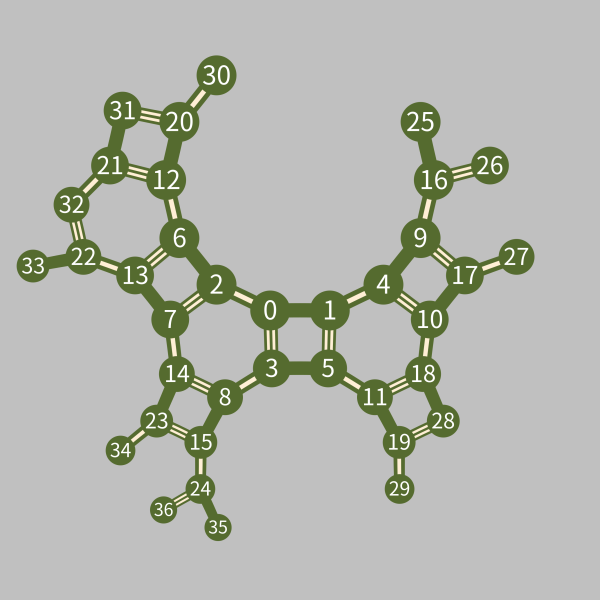1. 首先可以看出将顶点 0 关于三个镜面进行一次反射得到的新虚像是 1, 2, 3，它们对应的单词是 $L$ 中长度为 1 的 $s_0,s_1,s_2$
2. 将顶点 0 关于各个镜面进行两次反射得到的新虚像是 4, 5, 6, 7, 8，它们对应的单词是 $L$ 中长度为 2 的 $s_0s_1,s_0s_2,s_1s_0,s_1s_2,s_2s_1$
3. 所有顶点与初始顶点之间的最短路径的长度都不超过 5。
4. 从图中我们可以看出每个顶点对应的单词的规范表示。例如从 0 号顶点到 33 号顶点有两条最短路径：\begin{align*}&0\xrightarrow{\ s_1\ }2\xrightarrow{\ s_0\ }6\xrightarrow{\ s_2\ }13\xrightarrow{\ s_1\ }22\xrightarrow{\ s_0\ }33.\\ &0\xrightarrow{\ s_1\ }2\xrightarrow{\ s_2\ }7\xrightarrow{\ s_0\ }13\xrightarrow{\ s_1\ }22\xrightarrow{\ s_0\ }33. \end{align*} 只要按顺序连起来 (从左到右) 就可以得到 33 号顶点对应的两个单词：$s_1s_0s_2s_1s_0$$s_1s_2s_0s_1s_0$，它们都把 0 号顶点变为 33 号顶点，但是前者才是最短字典序表示。这一点也可以从顶点标号中看出来：从二号顶点开始两条路径分别去了 6 号和 7 号顶点，由于 6 号顶点在 $\mathcal{SL}(W)$ 中更小，因此这条路径必然才是 33 号顶点规范表示对应的路径。
5. 一个单词 $w=s_{i_0}s_{i_1}\cdots s_{i_n}$ 作用在 0 号顶点上 ($s_{i_n}$ 先作用，$s_{i_0}$ 在最后) 的结果是一条从 0 号顶点出发，标号依次为 $s_{i_0}, s_{i_1},\ldots,s_{i_n}$ 的路径 ($s_{i_0}$ 在先，$s_{i_n}$ 在最后)，与作用的顺序相反。

1. $T$ 的计算是一次性的，而且查表速度很快。不借助 $T$ 的话就要每条边都计算两次规范表示然后进行两次 $L$ 中的逐项比较查找。

2. $T$ 也同样用于计算面和胞腔。不借助 $T$ 的话就要对每个胞腔的每个顶点 (一个胞腔是一个多面体，可能有几十个顶点) 计算一次规范表示并逐项比较查找。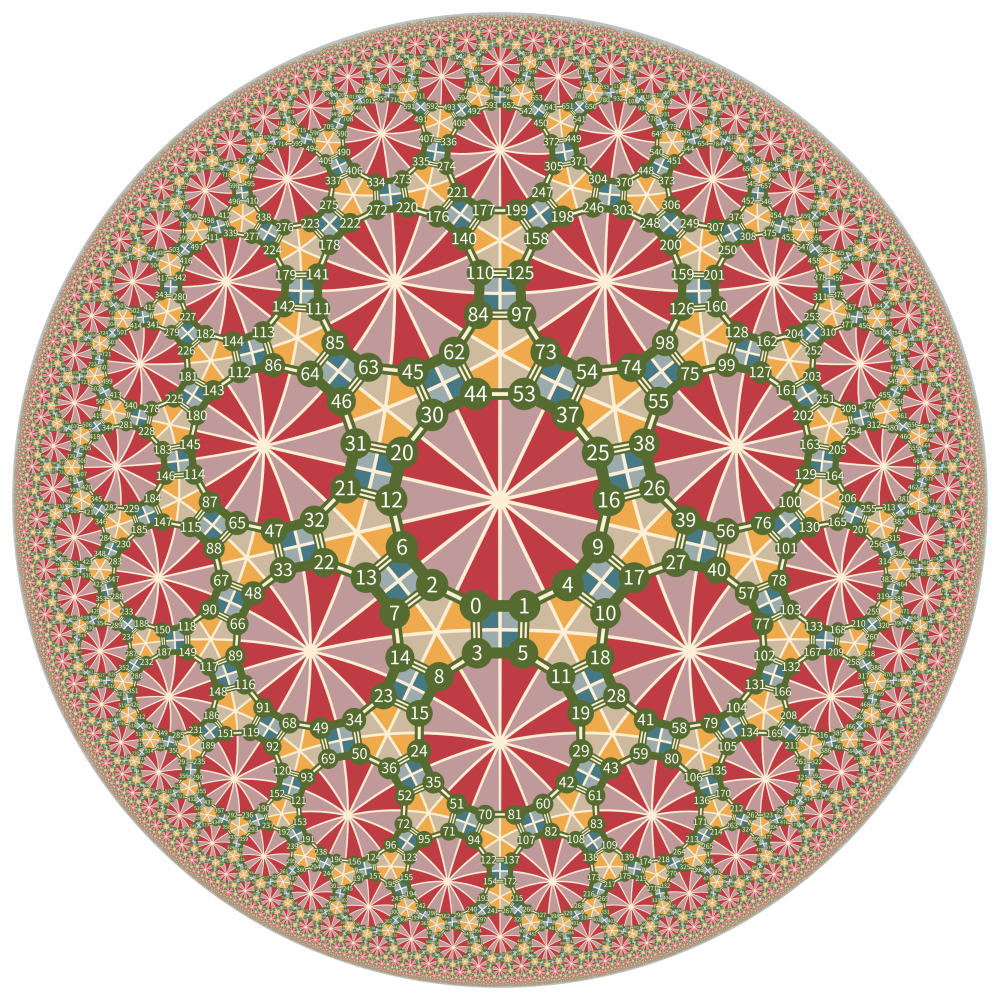# 算法运行效率

1. 计算群 $W$极小根部分。计算 Coxeter 群的极小根是计算 $\mathcal{SL}(W)$ 的有限状态机、计算群的规范表示所必须的重要步骤，而极小根的计算量依赖于 Coxeter 群的复杂度：对 $(p, q, r)$ 这样的 triangle 群，当 $p,q,r$ 都不大于 10 时计算还比较快 (双曲的话稍慢些，要花个几秒钟)，然而对 (19, 20, 21) 这样的群计算速度就很慢了。这一点与逆像素反射方法是不同的，逆像素反射法的计算量几乎不随群的变化而变化。

2. 计算乘法表 $T$。对于三维双曲密铺要生成一个效果较好的场景至少需要几十万个顶点，对这么多顶点计算乘法表非常耗时，内存和查找开销都很大。这种情形我采用的是有限状态机生成群元素结合传统的浮点数去重，一边计算一边输出以节省空间开销。

# 附录：对若干关键点的解释1. 如果 $\beta=\alpha_k$ 是第 $k$ 个极小根，则填入 $k$
2. 如果 $\beta$ 是一个负根 (此情形发生当且仅当 $\alpha_i$ 是第 $j$ 个单根)，则填入 -1
3. 如果 $\beta$ 是一个正根，但不是极小根，则填入 None

(7, 2, 3) 群的极小根反射表如下：

root $s_0$ $s_1$ $s_2$
0 -1 3 0
1 4 -1 5
2 2 5 -1
3 6 0 7
4 1 8 9
5 9 2 1
6 3 10 11
7 11 7 3
8 10 4 None
9 5 None 4
10 8 6 None
11 7 None 6

$W$ 的所有极小根之集为 $\Sigma$$\mathcal{W}$ 的有限状态机的状态都是 $\Sigma$ 的子集，状态之间的转移关系规定如下：

$S\xrightarrow{\ s_i\ } \{s_i\} \cup (s_i(S)\cup\{ s_i(\alpha_j),j<i\})\cap\Sigma.$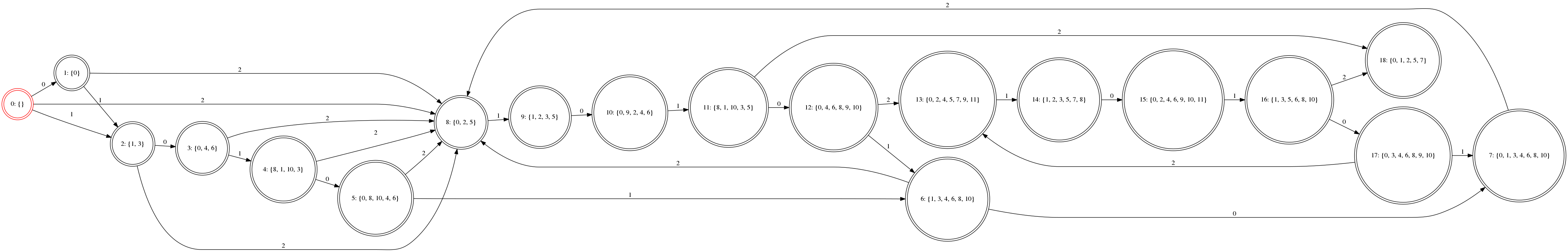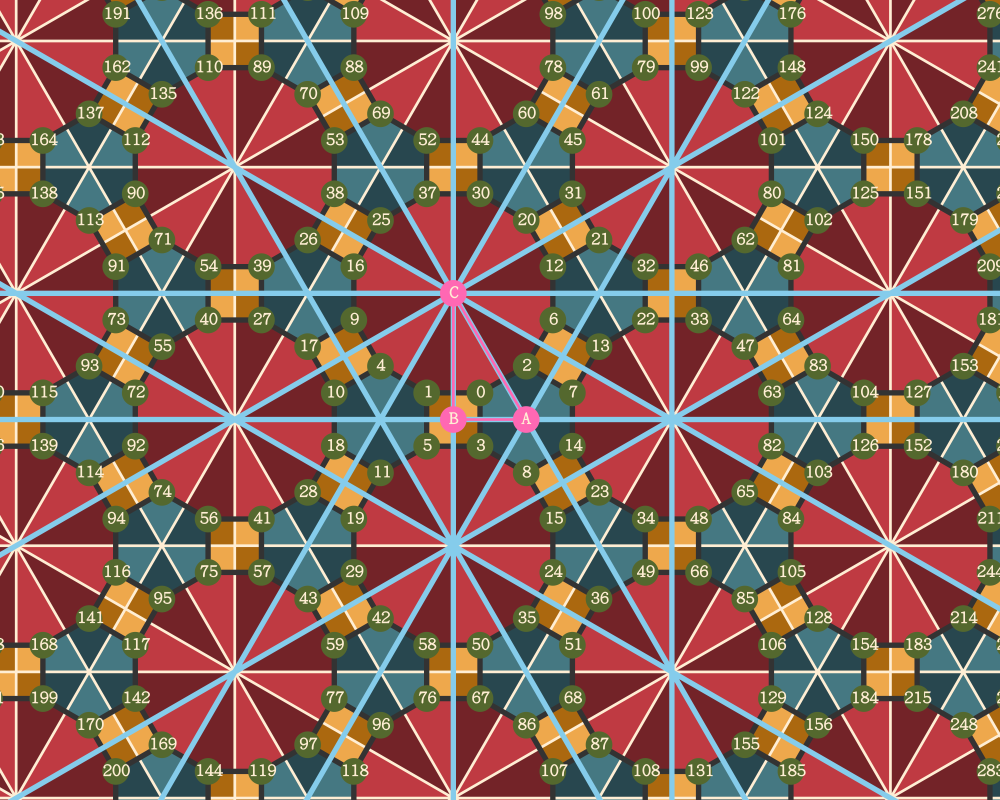1. 一个密铺的类型可以由其 Cartan 矩阵 $C=-\cos(\pi/M)$ 确定，是球面密铺当且仅当 $C$ 是正定的，是欧式密铺当且仅当 $C$ 是半正定的，是双曲密铺当且仅当 $C$ 是不定的，且 Coxeter-Dynkin 图的任何连通子图给出的密铺都是球面的或者欧式的。↩︎

2. Reflection Wroups and Coxeter Wroups, by James E. Humphreys.↩︎

|